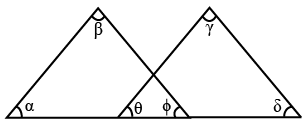# TS ICET 2014

Instructions

A question is followed by data in the form of two statements labelled as I and II. You must decide whether the data given in the statements are sufficient to answer the questions.

Question 11

# In the matrix $$A = \begin{bmatrix}-5 & 20 \\2 & -x \end{bmatrix}$$, what is the value of x ?I. A is singularII. A is symmetricQuestion 12

# What is the value of a + b ?I. $$a \neq b$$II. $$a^2 - b^2 - a - b$$Question 13

# Is the quadrilateral a square ?I. All the sides of the quadrilateral are of equal length.II. The diagonals of the quadrilateral area of equal length.Question 14

# For positve integers x, y and z, is the product xyz even ?I. x + y is odd.II. x + y + z is divisible by 7.Question 15

# What is the monthly salary of A ?I. A gets 15% more than B and B gets 10% less than C.II. C's monthly salary is ₹ 2,500.Question 16

# Among the real numbers a and b, is b a rational number ?I. a + b is a rational number.II. a - b is a rational number.Question 17

# How many persons are there in the library ?I. If 3 persons leave the library, then the library has less than 8 persons.II. If 3 persons enter the library, then it has more than 12 persons.Question 18

# In the figure given below, what is the value of $$\alpha + \beta + \gamma + \delta ?$$I. $$\alpha + \beta = \gamma + \delta$$II. $$\theta + \phi = 90^\circ$$Question 19

# How much is $$(x + y) : (x - y) ?$$I. x : y = 3 : 2II. x > 0, y > 0Question 20

# If p(x) is a polynomial, is (x - 2) a factor of $$p(2x^2 - 1) ?$$I. x - 1 is a factor of p(x).II. x - 7 is a factor of p(x).OR